In the following sequence :

(a) ${\mathrm{CH}}_{3}{\mathrm{CH}}_{2}{\mathrm{CH}}_{2}{\mathrm{NH}}_{2}$

(b) ${\mathrm{CH}}_{3}{\mathrm{CH}}_{2}{\mathrm{CH}}_{2}{\mathrm{CONHCH}}_{3}$

(c) ${\mathrm{CH}}_{3}{\mathrm{CH}}_{2}{\mathrm{CH}}_{2}{\mathrm{NHCOCH}}_{3}$

(d) ${\mathrm{CH}}_{3}{\mathrm{CH}}_{2}{\mathrm{CH}}_{2}{\mathrm{CONHCOCH}}_{3}$

Concept Questions :-

Acid Derivatives, Preparation and Properties
High Yielding Test Series + Question Bank - NEET 2020

Difficulty Level:

Carbonyl compounds can generally be converted to hydrocarbons by :

(a) H2/Pt

(b) LiAlH4

(c) ${\mathrm{N}}_{2}{\mathrm{H}}_{4}-\mathrm{KOH}/∆$

(d) ${\mathrm{K}}_{2}{\mathrm{Cr}}_{2}{\mathrm{O}}_{7}-{\mathrm{H}}_{2}{\mathrm{SO}}_{4}$

Concept Questions :-

Preparation & properties of Aldehydes and Ketones
High Yielding Test Series + Question Bank - NEET 2020

Difficulty Level:

Which statement about the aldol condensation is correct ?

(a) A Lewis acid is commonly used as a catalyst

(b) The initial step is probably the formation of a carbanion

(c) A Lewis base is employed to induce carbocation formation

(d) The carbon chain is lengthened through the elimination of 1 mole of water

Concept Questions :-

Preparation & properties of Aldehydes and Ketones
High Yielding Test Series + Question Bank - NEET 2020

Difficulty Level:

A compound gives a positive test with I2/NaOH and is extracted from benzene by saturated NaHSO3, It may be :

(a) ${\mathrm{CH}}_{3}{\left({\mathrm{CH}}_{2}\right)}_{4}\mathrm{CHO}$

(b) ${\mathrm{CH}}_{3}{\left({\mathrm{CH}}_{2}\right)}_{3}{\mathrm{COCH}}_{3}$

(c) ${\mathrm{CH}}_{3}{\mathrm{CH}}_{2}{\mathrm{COCH}}_{2}{\mathrm{CH}}_{3}$

(d) ${\mathrm{CH}}_{3}{\left({\mathrm{CH}}_{2}\right)}_{4}{\mathrm{CH}}_{2}\mathrm{OH}$

Concept Questions :-

Preparation & properties of Aldehydes and Ketones
High Yielding Test Series + Question Bank - NEET 2020

Difficulty Level: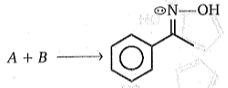Reactant (A) and (B) is:

(a)

(b)

(c)

(d)

Concept Questions :-

Preparation & properties of Aldehydes and Ketones
High Yielding Test Series + Question Bank - NEET 2020

Difficulty Level:

${\mathrm{CH}}_{3}-\stackrel{\stackrel{\mathrm{O}}{\mathrm{ll}}}{\mathrm{C}}-\mathrm{OH}\underset{∆}{\overset{\mathrm{Ca}{\left(\mathrm{OH}\right)}_{2}}{\to }}\left(\mathrm{A}\right)$

Product (A) is :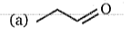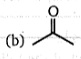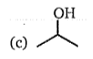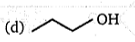Concept Questions :-

Carboxylic Acids
High Yielding Test Series + Question Bank - NEET 2020

Difficulty Level:

In which of the following compounds the methylenic hydrogens are the most acidic ?

(a) ${\mathrm{CH}}_{3}{\mathrm{COCH}}_{2}{\mathrm{CH}}_{3}$

(b) ${\mathrm{CH}}_{3}{\mathrm{CH}}_{2}{\mathrm{COOC}}_{2}{\mathrm{H}}_{5}$

(c) ${\mathrm{CH}}_{3}{\mathrm{CH}}_{2}\mathrm{CH}{\left({\mathrm{COOC}}_{2}{\mathrm{H}}_{5}\right)}_{2}$

(d) ${\mathrm{CH}}_{3}{\mathrm{COCH}}_{2}\mathrm{CN}$

Concept Questions :-

Preparation & properties of Aldehydes and Ketones
High Yielding Test Series + Question Bank - NEET 2020

Difficulty Level:

The presence of unsaturation in organic compounds can be tested with :

(a) Schiff's reagent

(b) Tollen's reagent

(c) Fehling's reagent

(d) Baeyer's reagent

Concept Questions :-

Preparation & properties of Aldehydes and Ketones
High Yielding Test Series + Question Bank - NEET 2020

Difficulty Level:

Compound (A) C6H12O is optically active. Compound (A) give negative Tollens test and positive test with 2-4 dinitro phenyl hydrazine. Identify A.

(a) ${\mathrm{CH}}_{3}-\stackrel{\stackrel{\mathrm{O}}{\parallel }}{\mathrm{C}}-{\mathrm{CH}}_{2}-\underset{\stackrel{\mathrm{l}}{{\mathrm{CH}}_{3}}}{\mathrm{CH}}-{\mathrm{CH}}_{3}$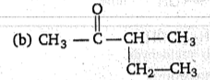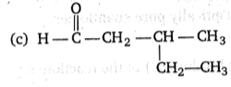(d) ${\mathrm{CH}}_{3}-\stackrel{\stackrel{\mathrm{O}}{\parallel }}{\mathrm{C}}-{\mathrm{CH}}_{2}-{\mathrm{CH}}_{2}-{\mathrm{CH}}_{2}-{\mathrm{CH}}_{3}$

Concept Questions :-

Preparation & properties of Aldehydes and Ketones
High Yielding Test Series + Question Bank - NEET 2020

Difficulty Level: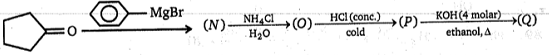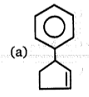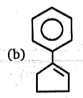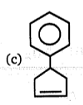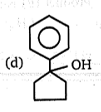Concept Questions :-

Preparation & properties of Aldehydes and Ketones